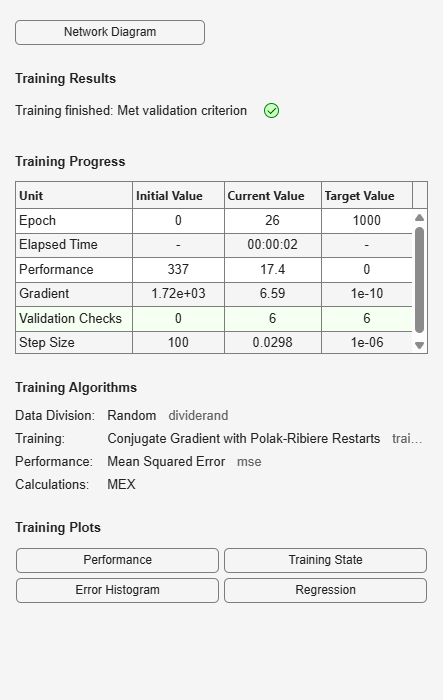# traincgp

## Syntax

```net.trainFcn = 'traincgp' [net,tr] = train(net,...) ```

## Description

`traincgp` is a network training function that updates weight and bias values according to conjugate gradient backpropagation with Polak-Ribiére updates.

`net.trainFcn = 'traincgp'` sets the network `trainFcn` property.

`[net,tr] = train(net,...)` trains the network with `traincgp`.

Training occurs according to `traincgp` training parameters, shown here with their default values:

 `net.trainParam.epochs` `1000` Maximum number of epochs to train `net.trainParam.show` `25` Epochs between displays (`NaN` for no displays) `net.trainParam.showCommandLine` `false` Generate command-line output `net.trainParam.showWindow` `true` Show training GUI `net.trainParam.goal` `0` Performance goal `net.trainParam.time` `inf` Maximum time to train in seconds `net.trainParam.min_grad` `1e-10` Minimum performance gradient `net.trainParam.max_fail` `6` Maximum validation failures `net.trainParam.searchFcn` `'srchcha'` Name of line search routine to use

Parameters related to line search methods (not all used for all methods):

 `net.trainParam.scal_tol` `20` Divide into `delta` to determine tolerance for linear search. `net.trainParam.alpha` `0.001` Scale factor that determines sufficient reduction in `perf` `net.trainParam.beta` `0.1` Scale factor that determines sufficiently large step size `net.trainParam.delta` `0.01` Initial step size in interval location step `net.trainParam.gama` `0.1` Parameter to avoid small reductions in performance, usually set to `0.1` (see `srch_cha`) `net.trainParam.low_lim` `0.1` Lower limit on change in step size `net.trainParam.up_lim` ` 0.5` Upper limit on change in step size `net.trainParam.maxstep` `100` Maximum step length `net.trainParam.minstep` `1.0e-6` Minimum step length `net.trainParam.bmax` `26` Maximum step size

## Network Use

You can create a standard network that uses `traincgp` with `feedforwardnet` or `cascadeforwardnet`. To prepare a custom network to be trained with `traincgp`,

1. Set `net.trainFcn` to `'traincgp'`. This sets `net.trainParam` to `traincgp`’s default parameters.

2. Set `net.trainParam` properties to desired values.

In either case, calling `train` with the resulting network trains the network with `traincgp`.

## Examples

collapse all

This example shows how to train a neural network using the `traincgp` train function.

Here a neural network is trained to predict body fat percentages.

```[x, t] = bodyfat_dataset; net = feedforwardnet(10, 'traincgp'); net = train(net, x, t);````y = net(x);`

collapse all

### Conjugate Gradient Backpropagation with Polak-Ribiére Updates

Another version of the conjugate gradient algorithm was proposed by Polak and Ribiére. As with the Fletcher-Reeves algorithm, `traincgf`, the search direction at each iteration is determined by

`${p}_{k}=-{g}_{k}+{\beta }_{k}{p}_{k-1}$`

For the Polak-Ribiére update, the constant βk is computed by

`${\beta }_{k}=\frac{\Delta {g}_{k-1}^{T}{g}_{k}}{{g}_{k-1}^{T}{g}_{k-1}}$`

This is the inner product of the previous change in the gradient with the current gradient divided by the norm squared of the previous gradient. See [FlRe64] or [HDB96] for a discussion of the Polak-Ribiére conjugate gradient algorithm.

The `traincgp` routine has performance similar to `traincgf`. It is difficult to predict which algorithm will perform best on a given problem. The storage requirements for Polak-Ribiére (four vectors) are slightly larger than for Fletcher-Reeves (three vectors).

## Algorithms

`traincgp` can train any network as long as its weight, net input, and transfer functions have derivative functions.

Backpropagation is used to calculate derivatives of performance `perf` with respect to the weight and bias variables `X`. Each variable is adjusted according to the following:

```X = X + a*dX; ```

where `dX` is the search direction. The parameter `a` is selected to minimize the performance along the search direction. The line search function `searchFcn` is used to locate the minimum point. The first search direction is the negative of the gradient of performance. In succeeding iterations the search direction is computed from the new gradient and the previous search direction according to the formula

```dX = -gX + dX_old*Z; ```

where `gX` is the gradient. The parameter `Z` can be computed in several different ways. For the Polak-Ribiére variation of conjugate gradient, it is computed according to

```Z = ((gX - gX_old)'*gX)/norm_sqr; ```

where `norm_sqr` is the norm square of the previous gradient, and `gX_old` is the gradient on the previous iteration. See page 78 of Scales (Introduction to Non-Linear Optimization, 1985) for a more detailed discussion of the algorithm.

Training stops when any of these conditions occurs:

• The maximum number of `epochs` (repetitions) is reached.

• The maximum amount of `time` is exceeded.

• Performance is minimized to the `goal`.

• The performance gradient falls below `min_grad`.

• Validation performance (validation error) has increased more than `max_fail` times since the last time it decreased (when using validation).

## References

Scales, L.E., Introduction to Non-Linear Optimization, New York, Springer-Verlag, 1985

## Version History

Introduced before R2006a